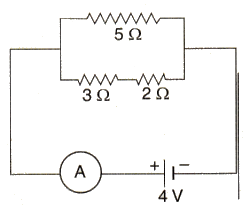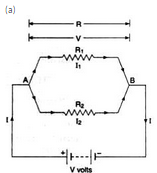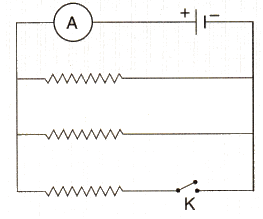Courses

# Solutions of Electricity (Page No- 42) - Physics By Lakhmir Singh, Class 10 Class 10 Notes | EduRev

## Class 10 : Solutions of Electricity (Page No- 42) - Physics By Lakhmir Singh, Class 10 Class 10 Notes | EduRev

The document Solutions of Electricity (Page No- 42) - Physics By Lakhmir Singh, Class 10 Class 10 Notes | EduRev is a part of the Class 10 Course Class 10 Physics Solutions By Lakhmir Singh & Manjit Kaur.
All you need of Class 10 at this link: Class 10

Question 34:
(a) With the help of a circuit diagram, obtain the relation for the equivalent resistance of two resistances connected in parallel.
In the circuit diagram shown below, find :
(i) Total resistance.
(ii) Current shown by the ammeter ASolution :Suppose total current flowing the circuit is I. then the current passing through resistance R1 wiII be I1 and current passing through resistance R2 will be l2 .

Total current = I = I1+I2

Let resultant resistance of this parallel combination is R. By applying the Ohm's law to the whole circuit. we get that I = V/R

Since the potential difference across the both the resistances is same. so applying the Ohm's law to each resistance we get that

I1 = V/R1

I= V/R2

Putting these eq in the above one, we get that

V/R = V/R1+V/R2

1/R = 1/R1+1/R2

If two resistance are connected in parallel than. the resultant resistance will be

1/R = 1/R1+1/R2

(b)

(i)Total reisitance = R

1/R=1/R1+1/R2

R= 3+2=5ohms

R1 = 5ohms

1/R=1/5+1/5

1/R=2/5

R=2.5ohms

Ili) Current flowing through the circuit

I = V/R = 4/(2.5)

= 1.6amps

Question 35:
(a) Explain with the help of a labelled circuit diagram, how you will find the resistance of a combination of three resistors of resistances R1, R2 and R3 joined in parallel.
(b) In the diagram shown below, the cell and the ammeter both have negligible resistance. The resistors are identical.With the switch K open, the ammeter reads 0.6 A. What will be the ammeter reading when the switch is closed ?
Solution :

Suppose total current flowing in the circuit is I. then the current passing through resistance R1 will be I1 Lcurrent passing through resistance R2 will be I2 and current passing through resistance Rwill bell I3.

Total current = 1 = I1+I2+I3

Let resultant resistance of this parallel combination is R. By applying the Ohm's law to the whole circuit. we get that

I=V/R

Since the potential difference across all the resistances is same. so applying the Ohm's law to each resistance we get that

l1 = V/R1
I2 = V/R2
I3 = V/R3

Putting these eqs. in the above one. we get

V/R = V/R1V/R2+ V/R3 .

1/R = 1/R1 + 1/R2+1/R3

If two resistance are connected in parallel, then the resultant resistance will be

1/R = 1/R1+1/R2+1/R3

(b) If switch is open. then only upper two resistances (connected in parallel) are in the circuit

Effective resistance is 1/Req = 1/R+1/R = 2/R

Req = R/2

So the current.I = VA(R/2) =0.6A (given)

V/R :0.3A

When the switch closes, the third resistance also comes in the circuit. The effective resistance of the circuit becomes R/3

Hence. Current 1= V/(R/3) = 3 (V/R) = 3 x 0.3 .0.9 A

Offer running on EduRev: Apply code STAYHOME200 to get INR 200 off on our premium plan EduRev Infinity!

94 docs

,

,

,

,

,

,

,

,

,

,

,

,

,

,

,

,

,

,

,

,

,

,

,

,

;